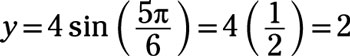##### Pre-Calculus: 1001 Practice Problems For Dummies (+ Free Online Practice)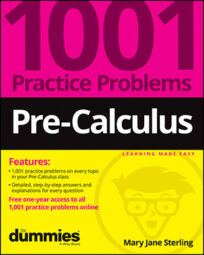You’ll work on graphing complex numbers. Polar coordinates are quite different from the usual (x, y) points on the Cartesian coordinate system. Polar coordinates bring together both angle measures and distances, all in one neat package. With the polar coordinate system, you can graph curves that resemble flowers and hearts and other elegant shapes.

You’ll work on complex numbers and polar coordinates in the following ways:

• Interpreting graphs of basic polar coordinates

• Graphing polar equations such as cardioids and lemniscates

When working with complex numbers and polar coordinates, some challenges will include the following:

• Moving in a counterclockwise direction when graphing polar coordinates

• Recognizing which ray to use when graphing negative and multiple angle measures

## Practice problems

1. Identify the point on the polar coordinate plane. Give your answer in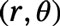form, where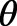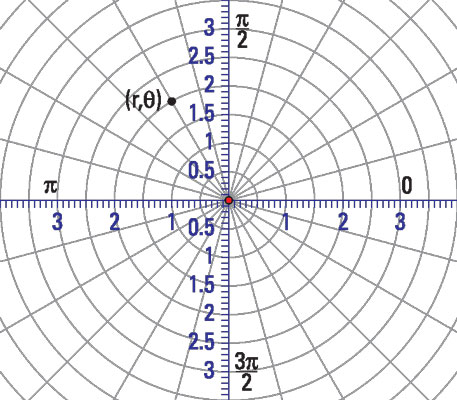Credit: Illustration by Thomson Digital

The point is on the 2-unit ring, so the radius is 2. You measure the angle from the positive x-axis in a counterclockwise direction. Each ray represents 15 degrees, so this point is on the ray representing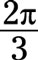The radius is 2, so the point is 2 units out from the origin.

2. Change the polar coordinates to rectangular coordinates.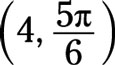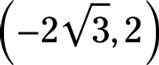You find the x coordinate with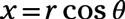so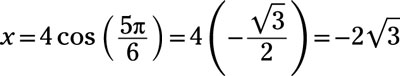You find the y coordinate with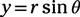so